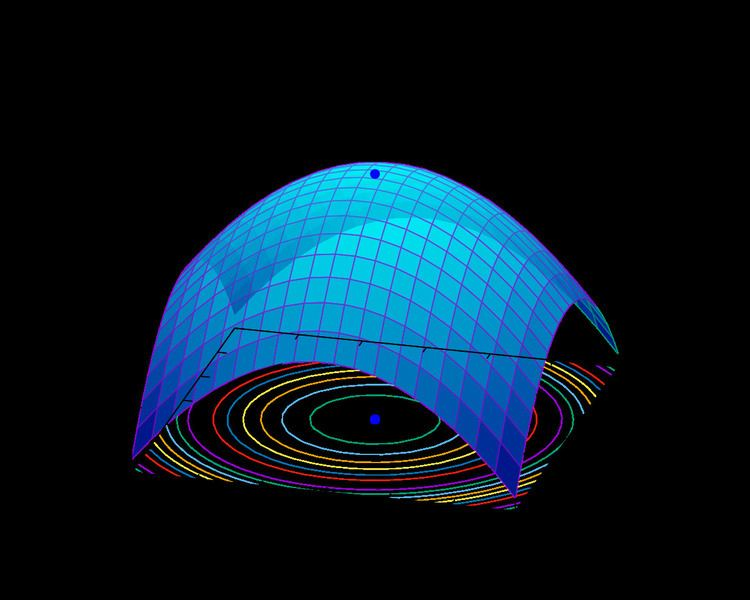# Iterative method

Updated on
Covid-19In computational mathematics, an iterative method is a mathematical procedure that generates a sequence of improving approximate solutions for a class of problems, in which the n-th approximation is derived from the previous ones. A specific implementation of an iterative method, including the termination criteria, is an algorithm of the iterative method. An iterative method is called convergent if the corresponding sequence converges for given initial approximations. A mathematically rigorous convergence analysis of an iterative method is usually performed; however, heuristic-based iterative methods are also common. In the problems of finding the root of an equation (or a solution of a system of equations), an iterative method uses an initial guess to generate successive approximations to a solution.

## Contents

In contrast, direct methods attempt to solve the problem by a finite sequence of operations. In the absence of rounding errors, direct methods would deliver an exact solution (like solving a linear system of equations A x = b by Gaussian elimination). Iterative methods are often the only choice for nonlinear equations. However, iterative methods are often useful even for linear problems involving a large number of variables (sometimes of the order of millions), where direct methods would be prohibitively expensive (and in some cases impossible) even with the best available computing power.

## Attractive fixed points

If an equation can be put into the form f(x) = x, and a solution x is an attractive fixed point of the function f, then one may begin with a point x1 in the basin of attraction of x, and let xn+1 = f(xn) for n ≥ 1, and the sequence {xn}n ≥ 1 will converge to the solution x. Here xn is the nth approximation or iteration of x and xn+1 is the next or n + 1 iteration of x. Alternately, superscripts in parentheses are often used in numerical methods, so as not to interfere with subscripts with other meanings. (For example, x(n+1) = f(x(n)).) If the function f is continuously differentiable, a sufficient condition for convergence is that the spectral radius of the derivative is strictly bounded by one in a neighborhood of the fixed point. If this condition holds at the fixed point, then a sufficiently small neighborhood (basin of attraction) must exist.

## Linear systems

In the case of a system of linear equations, the two main classes of iterative methods are the stationary iterative methods, and the more general Krylov subspace methods.

## Stationary iterative methods

Stationary iterative methods solve a linear system with an operator approximating the original one; and based on a measurement of the error in the result (the residual), form a "correction equation" for which this process is repeated. While these methods are simple to derive, implement, and analyze, convergence is only guaranteed for a limited class of matrices. Examples of stationary iterative methods are the Jacobi method, Gauss–Seidel method and the Successive over-relaxation method. Linear stationary iterative methods are also called relaxation methods.

## Krylov subspace methods

Krylov subspace methods work by forming a basis of the sequence of successive matrix powers times the initial residual (the Krylov sequence). The approximations to the solution are then formed by minimizing the residual over the subspace formed. The prototypical method in this class is the conjugate gradient method (CG). Other methods are the generalized minimal residual method (GMRES) and the biconjugate gradient method (BiCG).

## Convergence of Krylov subspace methods

Since these methods form a basis, it is evident that the method converges in N iterations, where N is the system size. However, in the presence of rounding errors this statement does not hold; moreover, in practice N can be very large, and the iterative process reaches sufficient accuracy already far earlier. The analysis of these methods is hard, depending on a complicated function of the spectrum of the operator.

## Preconditioners

The approximating operator that appears in stationary iterative methods can also be incorporated in Krylov subspace methods such as GMRES (alternatively, preconditioned Krylov methods can be considered as accelerations of stationary iterative methods), where they become transformations of the original operator to a presumably better conditioned one. The construction of preconditioners is a large research area.

## History

Probably the first iterative method for solving a linear system appeared in a letter of Gauss to a student of his. He proposed solving a 4-by-4 system of equations by repeatedly solving the component in which the residual was the largest.

The theory of stationary iterative methods was solidly established with the work of D.M. Young starting in the 1950s. The Conjugate Gradient method was also invented in the 1950s, with independent developments by Cornelius Lanczos, Magnus Hestenes and Eduard Stiefel, but its nature and applicability were misunderstood at the time. Only in the 1970s was it realized that conjugacy based methods work very well for partial differential equations, especially the elliptic type.

## References

Similar Topics
Berettas Island
Joe Parker (footballer)
Jack Shamama
Topics